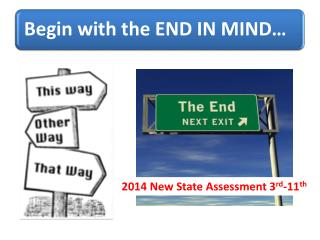# 2014 New State Assessment 3 rd -11 th - PowerPoint PPT PresentationDownload Presentation2014 New State Assessment 3 rd -11 th

2014 New State Assessment 3 rd -11 thDownload Presentation## 2014 New State Assessment 3 rd -11 th

- - - - - - - - - - - - - - - - - - - - - - - - - - - E N D - - - - - - - - - - - - - - - - - - - - - - - - - - -
##### Presentation Transcript

1. 2014 New State Assessment 3rd-11th

2. K

3. Read this – how much different is this than the K math you had to do?

4. Can we get to this level of writing with a letter sound a week curriculum?

5. st 1

6. Look at the amount of writing expected from a 1st grader?????? THOUGHTS…

7. This is an INFORMATIONAL/EXPLANATORY piece of writing. This research paper on Spain gives some very specific information.

8. NOTE the progressive levels of difficulty from A to B to 2 30 45 20 8 14 What diagnostic information might you derive from these answers? 59

9. What do we really want students to know? What does the equal sign really mean? = • It states a relationship between two quantities • If two quantities are equal, they represent the same amount • EQUALSmeans “THE SAME AS”

10. What do we really want students to know? What does “=“ mean? The equal sign? Equals means equals. First Grade 8.OA.7 : Understand the meaning of the equal sign, and determine if equations involving addition and subtraction are true or false.

11. Why should I reconsider how I teach “equality”? • A common misconception: • 3 + 4 = + 5 • 2 + = 3 + 4 • 3 + 4 = 2 + 1 1 Will leave empty or place a random number… or add all of the numbers Notice the lack of understanding of equality 9

12. Why should I reconsider how I teach “equality”? • Setting the foundation for … • 1/3 = 3/9 • 3/4 = 75% • (3 + 4) + 5 = 3 + (4 + 5) If a student doesn’t understand = in the previous problems they will struggle with advanced computations

13. nd 2

14. Does correctlyanswering this question tell you if they understand money? What is the total value of the coins? NO 25.MD.8

15. Does completing this question tell you if they understand money? If an item costs 50 cents how much more money would you need? Closer but not completely

16. Your mother sends you in to the store to buy something she wants. She tells you that the item costs somewhere between 1 cent and 99 cents. YES You only have pennies, nickels, dimes and quarters in the money jar. What is the least number of coins you can take with you to make change without going over \$1.00?

17. 2nd Grade

18. 2nd Grade -7.NBT.1 &12.NBT.4 & 11.NBT.3

19. Elementary Place Value Grade 2

20. rd 3

21. This is an example of an English Language Arts assessment question. Common Core Institute

22. Grade 3: Fractions As Numbers (3.NF.2) Which is closer to 1 on the number line, 4/5 or 5/4?

23. NOTICE the PROGRESSIVE nature of each question. 3rd Grade 14.NBT.3 & 3.OA.3

24. 3rd Grade 14.NBT.3 & 3.OA.3

25. Common Core Institute

26. th 4

27. At your table discuss: What era in history does this picture reflect? Justify your reasoning. Common Core Institute: Donna McCaw

28. At your table – solve this problem. 4th Grade: Common Core Institute

29. EXPERT LEVEL Common Core Institute

30. Practicioner LEVEL Common Core Institute

31. Common Core Institute

32. Common Core Institute

33. Common Core Institute

34. Common Core Institute

35. Here they must remember to use a wall of the house Common Core Institute

36. RUBRIC Common Core Institute

37. Common Core Institute

38. th 5

39. BEST is the focus point here Common Core Institute

40. Common Core Institute

41. Guiding Questions McDonald’s Claim Wikipedia reports that 8% of all Americans eat at McDonalds every day. In the US, there are approximately 310 million Americans and 12,800 McDonalds. The average McDonald’s store can serve 1,500 people a day. • What is the vocabulary that is critical for understanding the task? • What is the process that is necessary to solve the task? • What is the impact of this type of problem in your classroom Common Core Institute

42. Common Core Institute

43. th 6

44. Note card space is key! Common Core Institute

45. th 7